Documentation

# detrend

Subtract offset or trend from time-domain signals contained in `iddata` objects

## Syntax

``data_d = detrend(data)``
``data_d = detrend(data,Type)``
``[data_d,T_r] = detrend(___)``
``data_d = detrend(data,1,brkpt)``

## Description

`detrend` subtracts offsets or linear trends from time-domain input-output data represented in `iddata` objects. `detrend` either computes the trend data to subtract, or subtracts the trend that you specify.

For a more general detrending function that does not require `iddata` objects, see `detrend`.

example

````data_d = detrend(data)` computes and subtracts the mean value from each time-domain signal in `data`. The `iddata` objects `data_d` and `data` each contain input and output data originating from SISO, MIMO, or multiexperiment datasets.```

example

````data_d = detrend(data,Type)` subtracts the trend you specify in `Type`. You can specify a mean-value, linear, or custom trend.```

example

````[data_d,T_r] = detrend(___)` also returns the subtracted trend as a `TrendInfo` object, `T_r`. You can obtain `T_r` with any of the input-argument combinations in previous syntaxes.```

example

````data_d = detrend(data,1,brkpt)` computes and subtracts the piecewise-linear trends for data with segmented trends, using the breakpoints that you define with `brkpt`.The second argument, which corresponds to `Type`, must be `1`.With this syntax, you cannot retrieve the resulting piecewise-linear trend information as an output.```

## Examples

collapse all

Remove biases from steady-state signals in an `iddata` object by using `detrend` to compute and subtract the mean values of the input and output.

Load the input and output time series data `y2` and `u2`. Construct the `iddata` object `data2`, using` `the data and a sample time of 0.08 seconds.

```load dryer2 y2 u2 data2 = iddata(y2,u2,0.08);```

Use `detrend` to both compute the mean values and subtract them from input and output signals. Use the input argument `Tr` to store the computed trend information. Plot the original data and detrended data together.

```[data2_d,Tr] = detrend(data2); plot(data2,data2_d) legend('Original Data','Detrended Data')```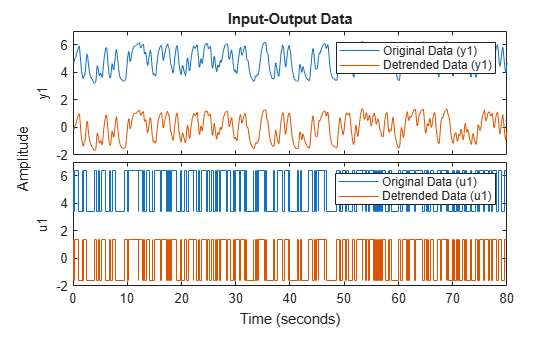The detrended data has shifted by about 5 units. Inspect `Tr` to obtain the precise mean values that `detrend` computed and subtracted. These values are returned in the `InputOffset` and `OutputOffset` properties.

`Tr`
```Trend specifications for data "data2" with 1 input(s), 1 output(s), 1 experiment(s): DataName: 'data2' InputOffset: 5.0000 OutputOffset: 4.8901 InputSlope: 0 OutputSlope: 0 ```

The mean of the original input is higher than the mean of the original output.

Remove the linear trend from a signal in an `iddata `object, and overlay the trendline on a before-and-after data plot.

Load and plot signal data from the file `lintrend2`. For this example, only output data is provided in `iddata` object `dataL`.

```load lintrend2 dataL plot(dataL,'b')```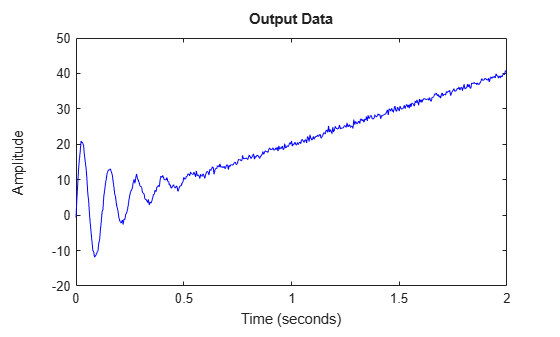The plot shows a clear linear trend in the data. Use `detrend `linear option (`Type` = 1) to subtract the trend from the data. `detrend` fits the data and determines the linear trend to subtract. Include the `TrendInfo` object `Tr` as an output argument so you can see what `detrend` subtracts.

`[dataL_d,Tr] = detrend(dataL,1);`

Plot the detrended data against the original data.

```hold on plot(dataL_d,'g') legend('Original','Detrended','Location','northwest')```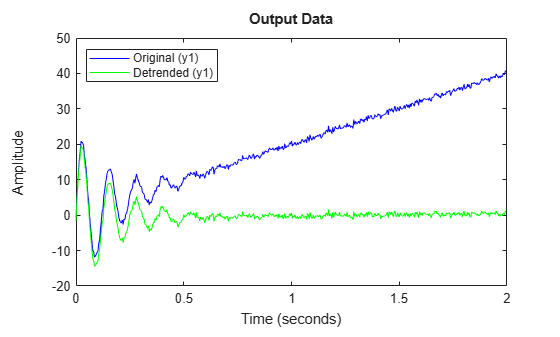The linear trend has been removed. Inspect `Tr` to get more information about the removed trend.

`Tr`
```Trend specifications for data "dataL" with 0 input(s), 1 output(s), 1 experiment(s): DataName: 'dataL' InputOffset: [1x0 double] OutputOffset: 0.8888 InputSlope: [1x0 double] OutputSlope: 19.3830 ```

The `OutputOffset` and the `OutputSlope` properties provide the parameters of the line that `detrend` removed. You can reconstruct this line, and then overlay it on the before-and-after data plot. The `SamplingInstants` property of `DataL `provides the timepoints associated with the data.

`m = Tr.OutputSlope`
```m = 19.3830 ```
`b = Tr.OutputOffset`
```b = 0.8888 ```
```t = dataL.SamplingInstants; TrLn = m*t+b; plot(t,TrLn,'r') legend('Original','Detrended','Trendline','Location','northwest')```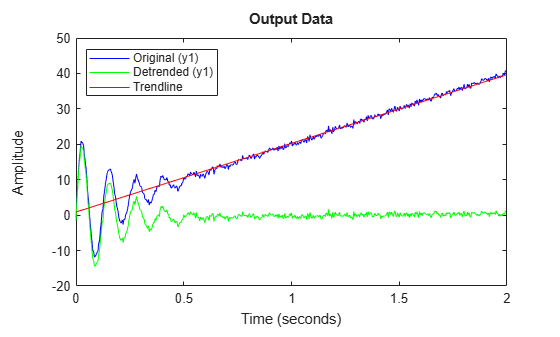Remove known offsets from an input-output signal pair contained in an `iddata object`.

`Detrend` can compute and subtract the mean values for input and output signals, resulting in zero-mean detrended signals. However, if you already know you have specific data offsets beforehand, you can have `detrend` subtract these from your signals instead. Specifying the offsets also allows you to retain a non-zero operating point in the `detrend` result.

Load SISO data containing vectors `u2` and `y2`. For this example, suppose that you know both signals have an offset of 4 from the expected operating point of 1. Combine these vectors into an `iddata object`, using a sample time of 0.08 seconds, and plot it.

```load dryer2 u2 y2 data = iddata(y2,u2,0.08); plot(data)```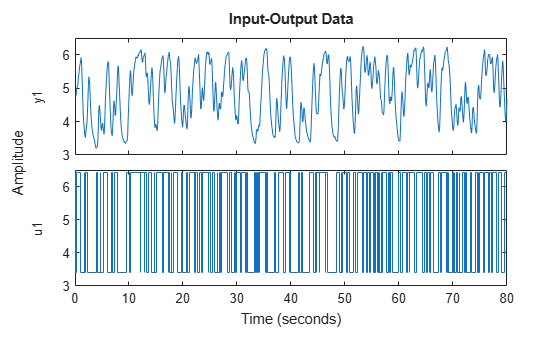The known offset of 4 (from operating point 1) is visible in the plots. You can construct a `TrendInfo` object to capture this offset, using the function `getTrend`.

Create the `TrendInfo` object, and then set its offset properties.

```T = getTrend(data); T.InputOffset = 4; T.OutputOffset = 4```
```Trend specifications for data "data" with 1 input(s), 1 output(s), 1 experiment(s): DataName: 'data' InputOffset: 4 OutputOffset: 4 InputSlope: 0 OutputSlope: 0 ```

Use `detrend` to subtract the offset from the data. Plot it alongside the original data.

```data_d = detrend(data,T); hold on plot(data_d) legend('Original','Detrended')```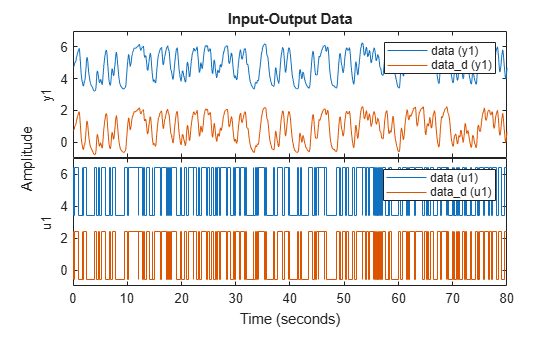The offset of 4 has been removed.

Detrend data with segmented piecewise-linear trends by specifying breakpoints to delimit the segments.

Most of the `detrend` syntaxes assume and compute a single trend for each of the signals. However, in some cases there are discontinuities in the linear trends, caused by test configuration changes, environmental conditions, or other influences. When the signal displays such segmentation, you can have `detrend` operate on the test segments independently. To do so, specify breakpoints in the `brkpt `input argument. These are the indices to the timepoints in the signal at which linear trends change slope.

You may know these breakpoints up front, based on changes that you know occurred during data collection. Alternatively, you may need to approximate them by inspecting the data itself.

Load the data, inspect its structure and contents, and plot it. This data consists of output data only in the `iddata` object `dataLb2.`

```load brkTrend dataLb2 dataLb2```
```dataLb2 = Time domain data set with 512 samples. Sample time: 0.00390625 seconds Outputs Unit (if specified) y1 ```
`plot(dataLb2)`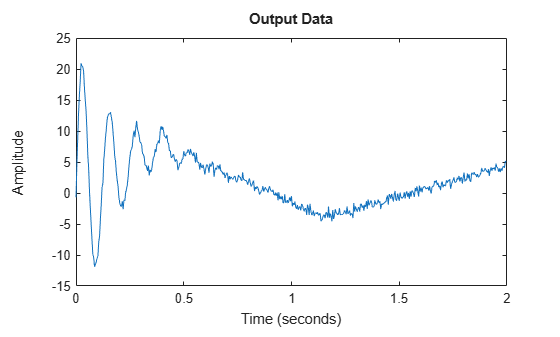For this example, the data has known breakpoints at indices [100 300]. Applying the sample time (property `Ts`), these breakpoints correspond to the actual timepoints as follows:

```brkpt=[100 300]; brkpt_time = brkpt*dataLb2.Ts```
```brkpt_time = 1×2 0.3906 1.1719 ```

Detrend the data using `brkp`t.

`dataLb2_d = detrend(dataLb2,1,brkpt);`

Plot the original and detrended data.

```plot(dataLb2,dataLb2_d) legend('Original Data','Detrended Data')```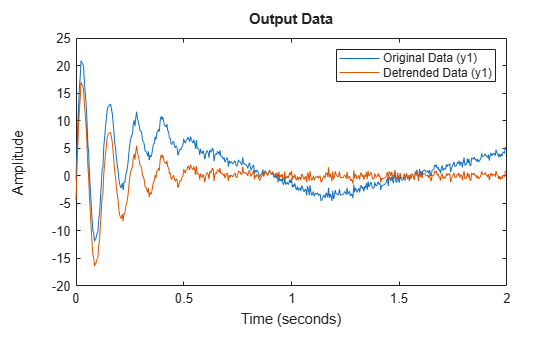The linear trend segments have been removed.

Apply a unique set of breakpoints to each experiment when you detrend a Multiexperiment dataset.

Experiments within a multiexperiment dataset may contain unique linear trending discontinuities. You can apply a unique set of breakpoints to each experiment by expressing them in a cell array.

Load the data, which consists of: ` `

• `datmult`, a multiexperiment `iddata` object containing three experiments (output only)

• `bp`n vectors, which provide known breakpoints for each experiment in the form of indices to timepoints

```load multiexpdt datmult bp1 bp2 bp3 datmult```
```datmult = Time domain data set containing 3 experiments. Experiment Samples Sample Time exp1 250 1 exp2 320 1 exp3 350 1 Outputs Unit (if specified) y1 ```
`bp1,bp2,bp3`
```bp1 = 1×2 50 200 ```
```bp2 = 100 ```
```bp3 = [] ```

Plot the data. There are significant differences among the streams, and they drift at different rates from zero mean.

```plot(datmult) legend```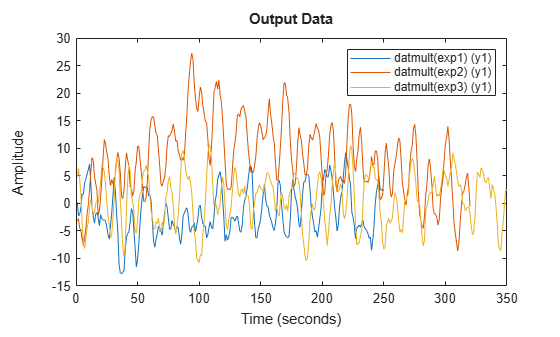For this set of experiments, it is known that there is unique trending for each run and unique discontinuities indicated by the `bp` vectors.

`detrend` can incorporate these unique characteristics if the `bp` information is provided as a cell array.

Construct the cell array.

`bpcell = {bp1;bp2;bp3}`
```bpcell=3×1 cell {1x2 double} {[ 100]} {0x0 double} ```

Apply `detrend `and plot the result, using the same scale as the original plot`.`

```datmult_d = detrend(datmult,1,bpcell); figure plot(datmult_d) axis([0,inf,-15,30]) legend```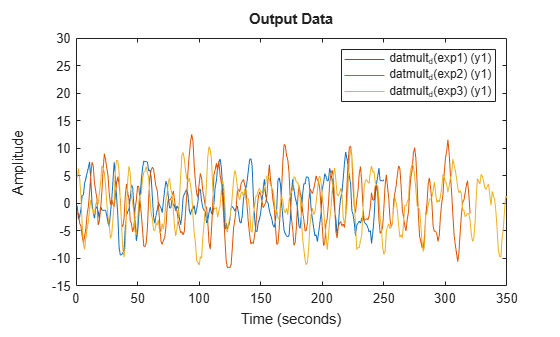The experimental data are now better aligned, and do not drift significantly away from zero mean.

Apply different trend types to the input and output signals contained in an` iddata` object.

`Detrend` assumes that the same type of trend applies to both input and output signals. In some cases, there may be a trend type that is present in only one signal. You can perform `detrend` individually on each signal by extracting the signals into separate `iddata` objects. Apply `detrend` to each object using its individual signal trend type. Then reassemble the results back into a single detrended `iddata` object.

Load, examine, and plot the data in `iodatab`.

```load septrend iodatab; iodatab```
```iodatab = Time domain data set with 1000 samples. Sample time: 0.08 seconds Outputs Unit (if specified) y1 Inputs Unit (if specified) u1 ```
```plot(iodatab) hold on```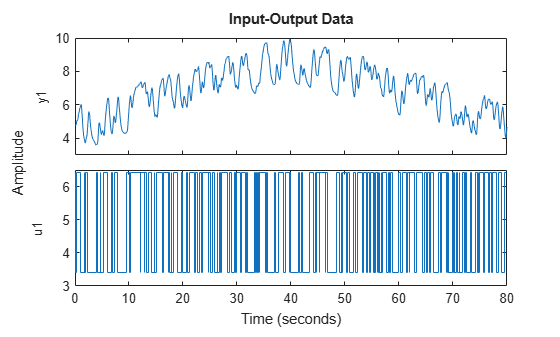Both input and output plots show a bias. However, the output plot also shows an inverted V-shape trend that is not present in the input data.

Separate the input data and the output data into separate objects for detrending, using the `iddata` general data-selection form (see Representing Time- and Frequency-Domain Data Using iddata Objects):

`data(samples,outputchannels,inputchannels)`

```idatab = iodatab(:,[],:); odatab = iodatab(:,:,[]);```

Remove the bias from the input data, using `detrend` to calculate and subtract the mean.

`idatab_d = detrend(idatab,0);`

Remove the bias and the inverted-V trend from the output data, using the midpoint index 500 as a breakpoint.

`odatab_d = detrend(odatab,1,500);`

Combine the detrended input and output data into a single `iddata` object.

`iodatab_d = [odatab_d,idatab_d];`

Overlay the detrended data on the original data.

```plot(iodatab_d) legend('original','detrended')```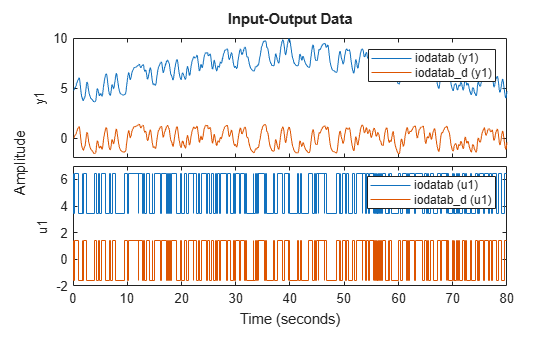The input and output data now contain neither bias nor V-shape trend.

## Input Arguments

collapse all

Specified as an `iddata` object containing one or more sets of time-domain signals. The `iddata` object can contain SISO, MIMO, or multiexperiment data. The signal sets can contain either input and output data, or output data only.

Specified as one of:

• 0 — Compute and subtract the mean value

• 1 — Compute and subtract the linear trend (least-squares fit)

• `TrendInfo` object — subtract the trend you specify in the `TrendInfo` object. Use `getTrend` to create a `TrendInfo` object. For an example, see Remove Specified Offsets from Signals.

Timepoint locations of trending discontinuities (breakpoints), specified as:

## Output Arguments

collapse all

Detrended signals, returned as an `iddata` object. Dimensions of the contents are the same as dimensions of the contents of `data`.

Trend data subtracted from `data` to produce `data_d`, returned as a `TrendInfo` object .

When you use `brkpt` to specify multiple trends, you cannot retrieve the computed trend data.